• MATLAB切比雪夫带通滤波器
万次阅读
2019-02-22 21:09:53

原始信号由5Hz，50Hz，110Hz三种频率的正弦信号构成，并含有直流分量。

原始信号为：y=sin(5*2*pi*x)+sin(50*2*pi*x)+sin(110*2*pi*x)+0.5;​

​使用通带为[10,100]Hz的切比雪夫滤波器，滤波后的信号时域曲线为：

​对原始信号和滤波信号作傅里叶变换，观察频谱的变化（左图为原始信号频谱，有图为滤波信号频谱）。

​对比图 3 可见，原始信号5Hz，110Hz的交流成分和直流成分都被滤掉了，只保留了50Hz交流成分，并且幅值吻合。

## 附切比雪夫II型带通滤波器程序

​function [X,Y]=Chebyshev2bp(x,y,fp,fs,Rp,Rs)

% fp 通带边界频率，单位Hz

% fs 阻带边界频率，单位Hz

% Rp 通带波纹，单位分贝（通常取值为1，该值越小通带幅值越平稳，但过渡带宽也越大）

% Rs 阻带衰减，单位分贝（从30附近试取，该值越大阻带幅值越小，但过渡带宽也越大）

wp=fp*2*pi;

ws=fs*2*pi;

[N,Wn]=cheb2ord(wp,ws,Rp,Rs,'s');  % 根据滤波器要求，求得滤波器最小阶数（计算也越慢）和截止频率（万永革 例5-17）

N

%%% 关于滤波器阶数N的说明

% 理论上，滤波器阶数越高越接近理想滤波器，但滤波器阶数达到一定值后再增加阶数，其逼近程度并不再显著提高，但计算量依然增加，所以理论上不需要无穷大的阶数

% 实际编程中，随着阶数无限升高，更根本的问题是计算机积累误差凸显，将使其与理想滤波器相去甚远，最终时域幅值严重失真，所以实际应用中滤波器阶数也不是越高越好

[z,p,k]=cheb2ap(N,Rs);  %设计Chebyshev II型原型低通滤波器（万永革 例5-8）

[b,a]=zp2tf(z,p,k);  % 将零点极点增益形式转换为传递函数形式

Wo=sqrt(Wn(1)*Wn(2));  % 计算中心点频率（万永革 例5-14）

Bw=Wn(2)-Wn(1);  % 计算频带宽度

[bt,at]=lp2bp(b,a,Wo,Bw);  % 频率转换。将模拟原先低通滤波器转换为带通滤波器

H=[tf(bt,at)];  % 在MATLAB中表示此滤波器（万永革 例5-13）

[Y,X]=lsim(H,y,x);

https://www.cnblogs.com/alimy/p/9140695.html

更多相关内容
• 切比雪夫滤波器MATLAB实现，可以直接用的子函数。 % 输入参数： % indata--输入信号 % Fs--采样频率 % pass1--通带上限 % pass2--通带下限 % stop1--阻带下限% stop2--阻带上限 % N--滤波器阶数 % 输出参数： % ...
• matlab m代码详细的实现了切比雪夫滤波器，有详细的代码说明，并且可以任意修改参数根据需求
• 提出了使用 MATLAB 设计巴特沃斯和切比雪夫滤波器的分析方法。 还计算了滤波器的阶数、传递函数以及各个滤波器的电容器和电感器的值。
• matlab切比雪夫仿真代码二维光谱-伽辽金电池热模型 该存储库包含用于实现二维切比雪夫谱伽辽金热模型并将其与有限元 (FEM) 模型进行比较的 Matlab 源代码。 此代码随附以下论文：Robert R. Richardson、Shi Zhao、...
• 框架已经写好 只需要更改 m q w0这些值 可求的S11 S12的曲线
• 在提交的 .m 文件中，我尝试比较在 16.148 MHz（16148 My Student ID）上运行的双线性滤波器和切比雪夫滤波器的响应。
• IIR切比雪夫II型滤波器（内含完整的MATLAB代码、CCS3.3软件仿真代码以及DSP实习报告）
• 1. 问题描述本人并非信号处理专业，仅在结构监测研究中遇到滤波问题...2. 技术背景在MATLAB中有很多种滤波器可供选择，本文仅介绍一笔者实现的滤波方式：切比雪夫滤波器。低通滤波的技术要点有：滤波器参数设置[n,Wp...

1. 问题描述

本人并非信号处理专业，仅在结构监测研究中遇到滤波问题，特总结常规的低通滤波技术，去除高频噪音。

由于环境的干扰因素，监测信号中总会包含噪音成分，影响信号处理过程，如下图：接收信号中出现很多“毛刺”，即为高频噪音，预期通过低通滤波器过滤处理。

2. 技术背景

在MATLAB中有很多种滤波器可供选择，本文仅介绍一笔者实现的滤波方式：切比雪夫滤波器。

低通滤波的技术要点有：

滤波器参数设置

[n,Wp]=cheb1ord(Wp,Ws,Rp,Rs); % Cheby1

[b,a]=cheby1(n,Rp,Wp);

freqz(b,a,2048,fs); % 查看设计滤波器的曲线

信号滤波运算

y = filter(b,a,x);

此处仅说明代码实现，理论问题不再说明。

3. 解决方案

滤波器参数的设置是有效滤波的关键，最重要的参数是确定滤波的范围：

通过频率$f_{pass}$

截止频率$f_{stop}$上图可以看出，原信号的频域范围主要在100~300kHz。故可以设置：

通过频率$f_{pass}= 300 kHz$

截止频率$f_{stop}= 500 kHz$

即过滤掉500 kHz以上的高频噪音。

4. 实施示例

4.1 数据读入

%% 数据读入

clc,clear,close all

[M,dt] = tools.getcsv(); % 读入csv信号和采样周期dt

fs = 1/dt; % 采样频率

t = M(:,1);

s = detrend(M(:,3)); % 去趋势的信号4.2 滤波参数设置

%% 参数设置

prompt0 = { % 对话框参数

'通过频率 f-pass(kHz)', 300

'截止频率 f-stop(kHz)', 500

'Passband ripple in decibels Rp',0.1

'衰减值Rs(Db)',30

};

dlg0.title = '滤波参数输入-马骋';

dlg0.save = 'lp';

para.f1 = para_input{1}*1e3;

para.f3 = para_input{2}*1e3;

para.rp = para_input{3};

para.rs = para_input{4};

para.fs = fs;

注：以上tools为笔者自定义函数工具箱。4.3 滤波器生成

%% cheby1低通滤波图示

para.type = 1; % 滤波器类型：切比雪夫-1

s_lp = tools.lowp(s,para); % 滤波可以看出，滤波器在频域300-500 kHz范围内逐渐衰减。

4.4 滤波效果

%% 处理信号绘图

figure

plot([t t],[s s_lp])

legend({'原始信号','低通滤波信号'})

title('cheby1低通滤波效果示例'),grid on

xlim([min(t) max(t)])

figure

subplot(211)

plot(t,s)

legend('原始信号'),grid on

xlim([min(t) max(t)])

subplot(212)

plot(t,s_lp)

legend('滤波信号'),grid on

xlim([min(t) max(t)])显然，滤波后的信号平滑很多。

5. 常见问题

滤波核心函数如下：

function y=lowp(x,para)

% 题目: 低通滤波器

% 输入:

% x -- 原始信号序列

% para.

% f1 -- 通带截止频率

% f3 -- 阻带截止频率

% rp -- 边带区衰减DB数设置

% rs -- 截止区衰减DB数设置

% fs -- 序列x的采样频率

% type-- 滤波器类型

% 输出：

% y -- 滤波后的信号

% 功能：

% 低通滤波，滤除高频噪音

% Cheby1

% Butterworth

% 注意：

% 通带或阻带的截止频率的选取范围是不能超过采样率的一半

% f1,f3的值都要小于fs/2

% rp=0.1;rs=30;%通带边衰减DB值和阻带边衰减DB值

% 作者: 未知

% 修改: 马骋

% 2016.04.21 @HIT

%% 参数输入

f1 = para.f1;

f3 = para.f3;

Rp = para.rp;

Rs = para.rs;

fs = para.fs;

%% 滤波器设计

Wp = f1/(fs/2); % 采用fs/2归一化,Nyquist frequency.

Ws = f3/(fs/2);

if para.type==1

[n,Wp]=cheb1ord(Wp,Ws,Rp,Rs); % Cheby1

[b,a]=cheby1(n,Rp,Wp);

freqz(b,a,2048,fs); % 查看设计滤波器的曲线

title(sprintf('n = %d Cheby1 Lowpass Filter',n))

xlim([0 f3])

else

[n,Wn] = buttord(Wp,Ws,Rp,Rs,'s'); % Butterworth

[b,a] = butter(n,Wn,'s'); % 计算滤波器系统函数分子分母多项式

[z,p,k] = butter(n,Wn);

sos = zp2sos(z,p,k);

freqz(sos,2048,fs)

title(sprintf('n = %d Butterworth Lowpass Filter',n))

xlim([0 f3])

end

%% 滤波

y = filter(b,a,x); % 对序列x滤波后得到的序列y

end % lowp

注：此函数中，仅切比雪夫-1滤波器测试成功，2型滤波器测试失败。

示例程序下载：本文用时 25 m

展开全文• MATLAB平台下，设计数字信号处理当中巴特沃斯和切比雪夫滤波器，包括低通、高通、带通和带阻等类型。
• ## 切比雪夫滤波器

千次阅读 2021-12-01 17:45:37
切比雪夫滤波器 切比雪夫滤波器，又名“车比雪夫滤波器”，是在通带或阻带上频率响应幅度等波纹波动的滤波器。 1、 切比雪夫滤波器传递函数 ∣Hn(ω)∣2=11+ϵ2Tn2ωω0|H_n(\omega)|^2 = \frac{1}{1+\epsilon^2{T_n...

# 切比雪夫滤波器

切比雪夫滤波器，又名“车比雪夫滤波器”，是在通带或阻带上频率响应幅度等波纹波动的滤波器。

1、 切比雪夫滤波器传递函数

∣ H n ( ω ) ∣ 2 = 1 1 + ϵ 2 T n 2 ω ω 0 |H_n(\omega)|^2 = \frac{1}{1+\epsilon^2{T_n}^2\frac{\omega}{\omega_0}}

其中 ω 0 \omega_0 为期望截至频率,n为滤波器阶数。

2、切比雪夫多项式

V n ( ω ω c ) = { c o s ( n ∗ a r c c o s ( ω ω c ) ) ∣ ω ω c ∣ ≤ 1 c o s h ( n ∗ a r c c o s h ( ω ω c ) ) ∣ ω ω c ∣ > 1 V_n(\frac{\omega}{\omega_c})=\left\{\begin{matrix}cos(n*arccos(\frac{\omega}{\omega_c}))\quad|\frac{\omega}{\omega_c}|\leq1 \\ cosh(n*arccosh(\frac{\omega}{\omega_c}))\quad|\frac{\omega}{\omega_c}|>1 \end{matrix}\right.

其中 ω c \omega_c 为期望截至频率,n为滤波器阶数。

下图为切比雪夫I型滤波器的振幅特性n为奇数n为偶数

2、特性

1)切比雪夫滤波器是在通带或阻带上频率响应幅度等波纹波动（通带平坦、阻带等波纹或是阻带平坦、通带等波纹）的滤波器，振幅特性在通带内是等波纹。

2)切比雪夫滤波器在过渡带比巴特沃斯滤波器的衰减快，但频率响应的幅频特性不如后者平坦。

3)切比雪夫滤波器和理想滤波器的频率响应曲线之间的误差最小，但是在通频带内存在幅度波动。

4)在通带（或称“通频带”）上频率响应幅度等波纹波动的滤波器称为“I型切比雪夫滤波器”，在阻带（或称“阻频带”）上频率响应幅度等波纹波动的滤波器称为“II型切比雪夫滤波器”

## python函数介绍

scipy.signal.iirfilter(N, Wn, rp=None, rs=None, btype='band', analog=False, ftype='butter', output='ba', fs=None)

参数：
N：int  过滤器的顺序。

rp：float, 可选参数
对于Chebyshev和椭圆滤波器，可在通带中提供最大纹波。(db)

rs：float, 可选参数
对于切比雪夫和椭圆滤波器，在阻带中提供最小的衰减。(db)

btype：{‘lowpass’, ‘highpass’, ‘bandpass’, ‘bandstop’}, 可选参数
过滤器的类型。默认值为‘lowpass’。

analog：bool, 可选参数
如果为True，则返回一个模拟滤波器，否则返回一个数字滤波器。

ftype：str, 可选参数
设计的IIR滤波器的类型：{Butterworth :‘butter’, Chebyshev I :‘cheby1’, Chebyshev II :‘cheby2’, Cauer/elliptic:‘ellip’, Bessel/Thomson:‘bessel’}

output：{‘ba’, ‘zpk’, ‘sos’}, 可选参数
输出类型：分子/分母(‘ba’)，pole-zero(‘zpk’)或second-order部分(‘sos’)。默认值为‘ba’。

fs：float  可选参数     数字系统的采样频率。
对于数字滤波器，Wn与fs的单位相同。默认情况下，fs为2 half-cycles /sample，因此将它们从0标准化为1，其中1是奈奎斯特频率。 (因此Wn在half-cycles /样本中。)

返回值：
b, a：ndarray，ndarray
IIR滤波器的分子(b)和分母(a)多项式。仅在以下情况下返回output='ba'。

z, p, k：ndarray，ndarray，float
IIR滤波器传递函数的零点，极点和系统增益。仅在以下情况下返回output='zpk'。

sos：ndarray
IIR滤波器的Second-order个部分表示。仅在以下情况下返回output=='sos'。


## python例子

生成一个从50 Hz到200 Hz的17th-order Chebyshev II模拟带通滤波器，并绘制频率响应：

from scipy import signal
import matplotlib.pyplot as plt
import numpy as np

b, a = signal.iirfilter(17, [2*np.pi*50, 2*np.pi*200], rs=60,
btype='band', analog=True, ftype='cheby2')
w, h = signal.freqs(b, a, 1000)
fig = plt.figure()
ax.semilogx(w / (2*np.pi), 20 * np.log10(np.maximum(abs(h), 1e-5)))
ax.set_title('Chebyshev Type II bandpass frequency response')
ax.set_xlabel('Frequency [Hz]')
ax.set_ylabel('Amplitude [dB]')
ax.axis((10, 1000, -100, 10))
ax.grid(which='both', axis='both')
plt.show()最后附上matlab实现代码，代码来源Blog

%  Cheby1Filter.m
%  切比雪夫Ⅰ型滤波器的设计
%

clear;
close all;
clc;

fs = 1000; %Hz 采样频率
Ts = 1/fs;
N  = 1000; %序列长度
t = (0:N-1)*Ts;
delta_f = 1*fs/N;
f1 = 50;
f2 = 100;
f3 = 200;
f4 = 400;
x1 = 2*0.5*sin(2*pi*f1*t);
x2 = 2*0.5*sin(2*pi*f2*t);
x3 = 2*0.5*sin(2*pi*f3*t);
x4 = 2*0.5*sin(2*pi*f4*t);
x = x1 + x2 + x3 + x4; %待处理信号由四个分量组成

X = fftshift(abs(fft(x)))/N;
X_angle = fftshift(angle(fft(x)));
f = (-N/2:N/2-1)*delta_f;

figure(1);
subplot(3,1,1);
plot(t,x);
title('原信号');
subplot(3,1,2);
plot(f,X);
grid on;
title('原信号频谱幅度特性');
subplot(3,1,3);
plot(f,X_angle);
title('原信号频谱相位特性');
grid on;

%设计一个切比雪夫低通滤波器,要求把50Hz的频率分量保留,其他分量滤掉
wp = 55/(fs/2);  %通带截止频率,取50~100中间的值,并对其归一化
ws = 90/(fs/2);  %阻带截止频率,取50~100中间的值,并对其归一化
alpha_p = 3; %通带允许最大衰减为 db
alpha_s = 40;%阻带允许最小衰减为 db
%获取阶数和截止频率
[N1, wc1] = cheb1ord(wp, ws, alpha_p, alpha_s);
%获得转移函数系数
[b, a] = cheby1(N1,alpha_p,wc1,'low');
%滤波
filter_lp_s = filter(b,a,x);
X_lp_s = fftshift(abs(fft(filter_lp_s)))/N;
X_lp_s_angle = fftshift(angle(fft(filter_lp_s)));
figure(2);
freqz(b,a); %滤波器频谱特性
figure(3);
subplot(3,1,1);
plot(t,filter_lp_s);
grid on;
title('低通滤波后时域图形');
subplot(3,1,2);
plot(f,X_lp_s);
title('低通滤波后频域幅度特性');
subplot(3,1,3);
plot(f,X_lp_s_angle);
title('低通滤波后频域相位特性');

%设计一个高通滤波器,要求把400Hz的频率分量保留,其他分量滤掉
wp = 350/(fs/2);  %通带截止频率,取200~400中间的值,并对其归一化
ws = 380/(fs/2);  %阻带截止频率,取200~400中间的值,并对其归一化
alpha_p = 3; %通带允许最大衰减为  db
alpha_s = 20;%阻带允许最小衰减为  db
%获取阶数和截止频率
[N2, wc2] = cheb1ord( wp , ws , alpha_p , alpha_s);
%获得转移函数系数
[b, a] = cheby1(N2,alpha_p,wc2,'high');
%滤波
filter_hp_s = filter(b,a,x);
X_hp_s = fftshift(abs(fft(filter_hp_s)))/N;
X_hp_s_angle = fftshift(angle(fft(filter_hp_s)));
figure(4);
freqz(b,a); %滤波器频谱特性
figure(5);
subplot(3,1,1);
plot(t,filter_hp_s);
grid on;
title('高通滤波后时域图形');
subplot(3,1,2);
plot(f,X_hp_s);
title('高通滤波后频域幅度特性');
subplot(3,1,3);
plot(f,X_hp_s_angle);
title('高通滤波后频域相位特性');

%设计一个带通滤波器,要求把50Hz和400Hz的频率分量滤掉,其他分量保留
wp = [65 385] / (fs/2);  %通带截止频率,50~100、200~400中间各取一个值,并对其归一化
ws = [75 375] / (fs/2);  %阻带截止频率,50~100、200~400中间各取一个值,并对其归一化
alpha_p = 3; %通带允许最大衰减为  db
alpha_s = 20;%阻带允许最小衰减为  db
%获取阶数和截止频率
[N3, wn] = cheb1ord( wp , ws , alpha_p , alpha_s);
%获得转移函数系数
[b, a] = cheby1(N3,alpha_p,wn,'bandpass');
%滤波
filter_bp_s = filter(b,a,x);
X_bp_s = fftshift(abs(fft(filter_bp_s)))/N;
X_bp_s_angle = fftshift(angle(fft(filter_bp_s)));
figure(6);
freqz(b,a); %滤波器频谱特性
figure(7);
subplot(3,1,1);
plot(t,filter_bp_s);
grid on;
title('带通滤波后时域图形');
subplot(3,1,2);
plot(f,X_bp_s);
title('带通滤波后频域幅度特性');
subplot(3,1,3);
plot(f,X_bp_s_angle);
title('带通滤波后频域相位特性');

%设计一个带阻滤波器,要求把50Hz和400Hz的频率分量保留,其他分量滤掉
wp = [65 385 ] / (fs/2);  %通带截止频率?,50~100、200~400中间各取一个值,并对其归一化
ws = [75 375 ] / (fs/2);  %阻带截止频率?,50~100、200~400中间各取一个值,并对其归一化
alpha_p = 3; %通带允许最大衰减为  db
alpha_s = 20;%阻带允许最小衰减为  db
%获取阶数和截止频率
[N4, wn] = cheb1ord( wp , ws , alpha_p , alpha_s);
%获得转移函数系数
[b, a] = cheby1(N4,alpha_p,wn,'stop');
%滤波
filter_bs_s = filter(b,a,x);
X_bs_s = fftshift(abs(fft(filter_bs_s)))/N;
X_bs_s_angle = fftshift(angle(fft(filter_bs_s)));
figure(8);
freqz(b,a); %滤波器频谱特性
figure(9);
subplot(3,1,1);
plot(t,filter_bs_s);
grid on;
title('带阻滤波后时域图形');
subplot(3,1,2);
plot(f,X_bs_s);
title('带阻滤波后频域幅度特性');
subplot(3,1,3);
plot(f,X_bs_s_angle);

展开全文• 基于MATLAB切比雪夫低通滤波器设计.pdf
• 切比雪夫滤波器属于IIR滤波器，即无限脉冲响应滤波器，利用上一时刻的输出信号，进行递归计算，它可以使用相同阶的滤波器实现更陡峭的增益变化.，从而获得更少的阶数，也就意味着减少计算时间。 滤波器的工作目的是...

# Matlab基础——切比雪夫I型滤波器（二）

切比雪夫滤波器属于IIR滤波器，即无限脉冲响应滤波器，利用上一时刻的输出信号，进行递归计算，它可以使用相同阶的滤波器实现更陡峭的增益变化.，从而获得更少的阶数，也就意味着减少计算时间。
滤波器的工作目的是在频域去除非有用频率信号，但是 滤波器是在时域进行工作的(图3). 滤波器以时域信号为输入，修改频率内容，得到新的时域信号.。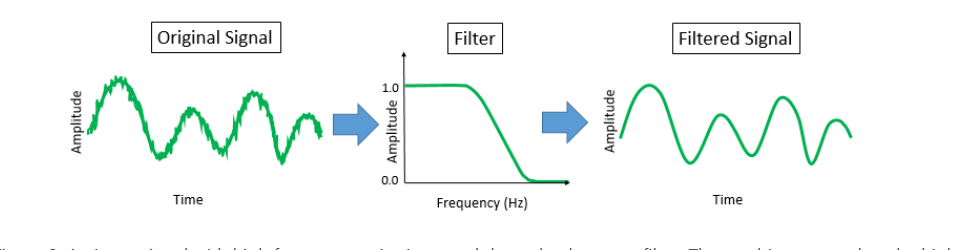## 频率响应函数freqz()、angle()和phasez()—查看滤波器效果

①freqz() 用来展示滤波器的频域响应
语法：freqz(B,A) B、A为滤波器系数
‘ freqz（B，A，n，fs）显示频率下的频域响应。——用此图较为简单观察。
如图一所示：通带随频率的衰减幅度（Rp=3dB/hz）。阻带的下降幅度（Rs=40dB/hz）

## 一般来说，理想滤波器，应该是通带衰减较少、阻带下降较陡。

② [h,w]=freqz(b, a);具备返回值时，h：代表响应的幅值
[h,f]=freqz(b, a，n，fs) f代表的频率
绘图：
————频域图
plot(f,20
log10(abs(h)))；xlabel(‘Normalized frequency(Hz)’), ylabel(‘Amplitude(dB)’)；
————相位图
[phi, w1]=phasez(b, a) %phasez返回值phi以弧度制表示，w为角频率
plot(w/pi, phi
180/pi), grid

## cheb1ord函数的Rp与Rs参数

Rp是指通带的衰减幅度，Rs是阻带的下降速度，单位都是dB
例程给的参数为Rp=3，Rs=40；
下面是参数不同时候的频率响应图像

### Rp

图一：Rp=3，Rs=40。下降曲线顺滑，频域速率由-3dB随频率下降。相位图则是-40dB下降，但图中在25hz才能达到。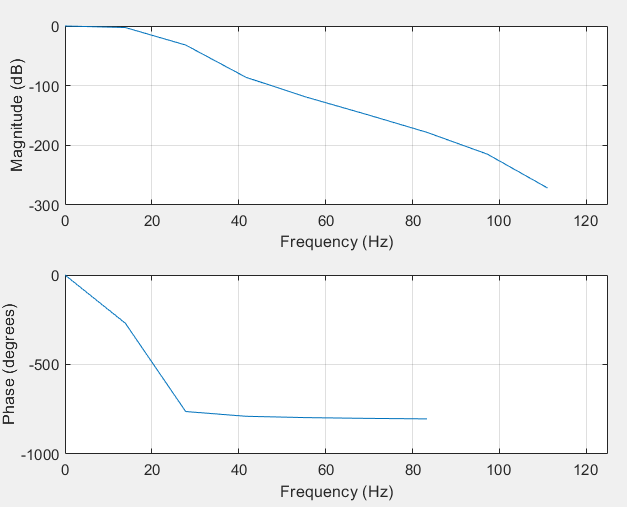图二：Rp=100，Rs=40.程序出错，因此Rp不能太大，此时Rp=30.
相位延迟加大且下降速度较慢。但通道衰减较弱，且滤波后信号保留信息较少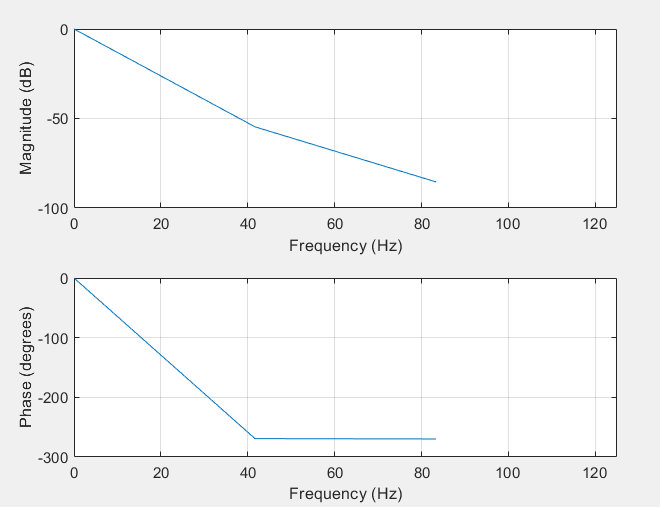图三 Rp=0.5，Rs=40。此时，通道几乎完全没有滤波。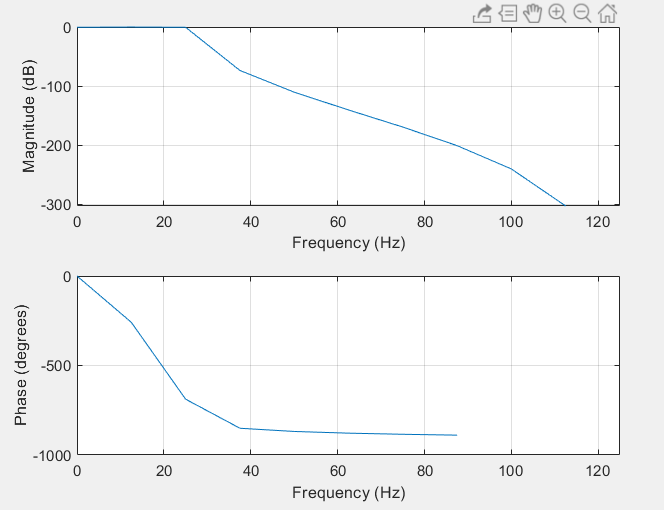### Rs

图一：Rp=3，Rs=400，相位发生偏转，且抖动严重，表示不稳定，无滤波结果
图二，Rp=3，Rs=4，若小于4则报错。此时滤波效果较差，阻带存在部分干扰信号。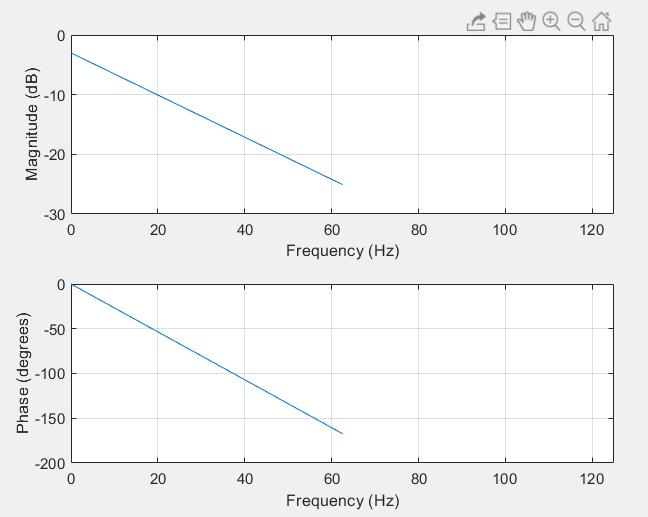## 滤波器在时域与频域

原信号时域图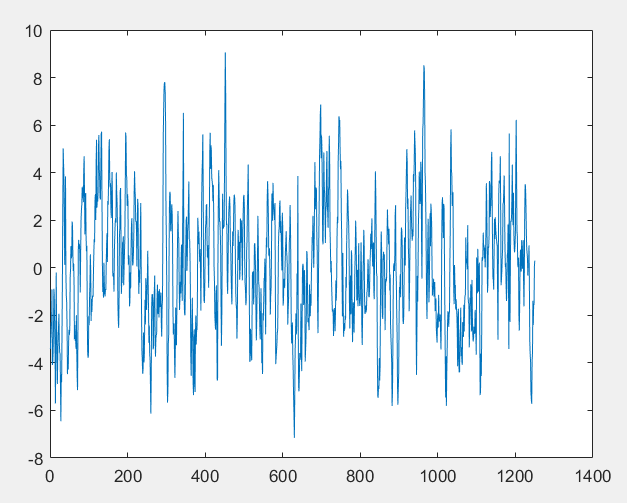频域表现为：（只是简单用到傅里叶变化，应该用短时傅里叶/分数阶傅里叶、小波变换）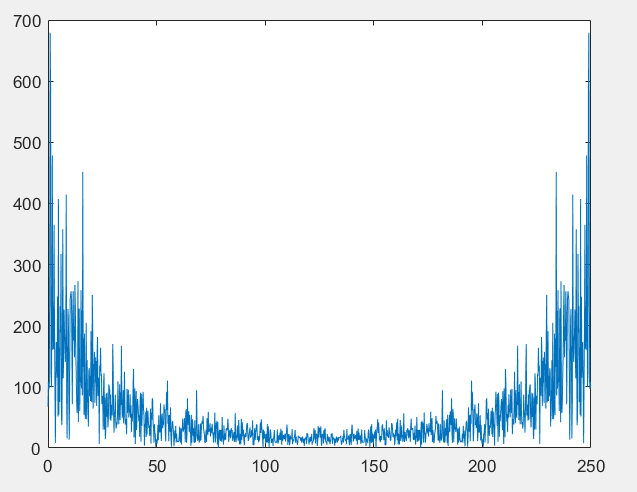① 滤波器工作在频域 存在幅值与相位的变化，与原信号存在较大偏差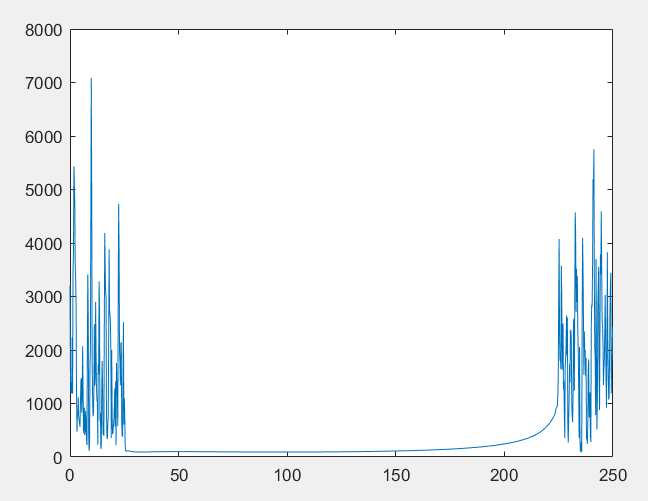②滤波器工作在时域，滤波效果较好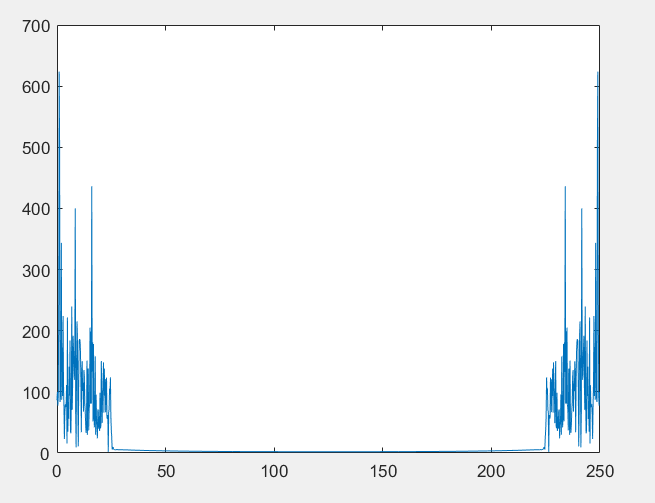matlab代码如下

clear all
clc
fs=250;

x(:)=eeg(1,1,:,1);
figure(1)
plot(x)

%m=fft(x);
%mag=abs(m);
%fm=(0:length(m)-1)'*fs/length(m)
%figure(2)
%plot(fm,mag)

[n,Wn]=cheb1ord(50/fs,60/fs,3,40);
[B,A]=cheby1(n,3,Wn);

x1=filtfilt(B,A,x)

y=fft(x1);
f=(0:length(y)-1)'*fs/length(y)

figure(3)
plot(f,abs(y))


## 参考文献

https://blog.csdn.net/weixin_42765703/article/details/104844894

## Matlab基础——切比雪夫Ⅰ型滤波器（一）

展开全文• Matlab——切比雪夫I型滤波器 cheb1ord 、 chey1 and freqz 切比雪夫Ⅰ型滤波器在通带或阻带上频率响应幅度等波纹波动的...切比雪夫滤波器和理想滤波器的频率响应曲线之间的误差最小，但是在通频带内存在幅度波动。
• II型切比雪夫滤波器 在阻带（或称“阻频带”）上频率响应幅度等波纹波动的滤波器称为“II型切比雪夫滤波器”。 也称倒数切比雪夫滤波器，较不常用，因为频率截止速度不如I型快，也需要用更多的电子元件。II型...数字信号处理
• 处理的是心电信号，采样频率为360hz,这个该怎么写matlab代码啊，我看了别人的，但是不理解通带截止频率，阻带截止频率，这个4和22该放在哪里,恳请大佬指导一下  </p>开发语言
• 摘要:数字滤波器因其具有...在设计切比雪夫I型数字滤波器的过程中，主要利用MATLAB软件，经过归一化处理，同时调用cheby1和cheb1ord语句来实现低通、高通等4种不同类型的切比雪夫I型数字滤波器，并对实验参数进行验...
• MATLAB实现FIR ，在MATLAB中利用滤波器设计、分析工具( FDATOOL) ,根据指定的滤波器性能快速设计一个FIR ,再把滤波器系数以头文件形式导入CCS 中,头文件中MATLAB实现FIR 数字滤波器滤波器阶数和系数数组,在MATLAB...
• 目录 TOC \o "1-2" \h \z \u 1 课题综述 1 1.1 课题来源 1 1.2 预期目标 1 1.3 面对的问题 1 1.4 需要解决的关键技术 1 2 系统分析 2 2.1 ...3 3.1 巴特沃斯低通滤波特性(MATLAB) 3 3.2 巴特沃斯高通滤波特性(MATLAB
• 四阶对称广义切比雪夫滤波器详细计算过程【matlab】.pdf
• matlab滤波器切比雪夫为例）实验 数字滤波器的定义 所谓数字滤波器，是指输入、输出均为数字信号，通过数值运算改变输入信号所含频率成分的相对比例，或者滤除某些频率成分的数字器件或程序。 数字滤波器的分类 ...
• 切比雪夫滤波器，又名“车比雪夫滤波器”，是在通带或阻带上频率响应幅度等波纹波动的滤波器。切比雪夫滤波器来自切比雪夫分布，以“切比雪夫”命名，是用以纪念俄罗斯数学家巴夫尼提·列波维其·切比雪夫。 ...
• 切比雪夫Ⅰ型滤波器特点： 1、幅度特性是在一个频带内（通带或阻带）范围内具有等波纹特性； 2、Ⅰ型在通带范围内是等波纹的，在阻带范围内是单调的。 测试代码： % Cheby1Filter.m % 切比雪夫Ⅰ型滤波器的...
• 以下两个滤波器都是切比雪夫I型数字滤波器，不是巴特沃尔滤波器，请使用者注意！1.低通滤波器使用说明：将下列代码幅值然后以m文件保存，文件名要与函数名相同，这里函数名：lowp。function y=lowp(x,f1,f3,rp,rs,Fs...
• MATLAB中，cheblap函数用于设计切比雪夫 I 型低通滤波器。该函数的调用方法为 [z, p,k] = cheb1ap(n, rp) 其中,n为滤波器的阶数,rp为通带的幅度误差。返回值分别为滤波器的零点、极点和增益。 例 设计切比雪夫I型...数字信号处理
• 常见滤波器简要介绍及Matlab实现 简要收集关于“椭圆、切比雪夫、巴特沃斯、贝塞尔”滤波器的使用与特点，不完善之处还请提出意见与谅解！ 1.椭圆滤波器EllipticFilter ①椭圆滤波器又称考尔滤波器（Cauer ...
• matlab编写的IIR低通切比雪夫滤波器及其滤波过程。自己写的，简单易懂。。。对初学者很有用。。。需要设计相关程序的话可以借鉴一下
• 用C语言改写的Matlab中的几个函数，适用于嵌入式设备。其中 void dwt_filter(double *inp,double *outa,double *outd, char *name,int len)； char hampel(float *Y1, float *uhy, unsigned int len, unsigned int ...
• 本资源包含一个matlab代码文件和两个音乐信号，通过切比雪夫一型滤波器滤除高频噪声，同时用均值滤波滤除白噪声
• IIR数字滤波器是数字信号处理的重要工具之一。利用Matlab信号处理工具箱和使用切比雪夫法设计IIR数字滤波器,并进行仿真。仿真结果表明,设计过程简单方便。...

# matlab切比雪夫滤波器matlab 订阅